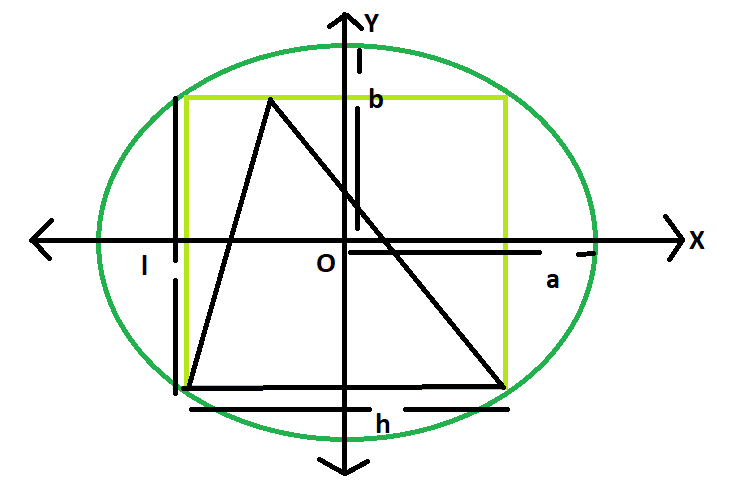Open in App
Not now

# Area of a triangle inscribed in a rectangle which is inscribed in an ellipse

• Last Updated : 28 Jun, 2022

Given here is an ellipse with axes length 2a and 2b, which inscribes a rectangle of length l and breadth h, which in turn inscribes a triangle.The task is to find the area of this triangle.
Examples:

```Input: a = 4, b = 3
Output: 12

Input: a = 5, b = 2
Output: 10```Approach
We know the Area of the rectangle inscribed within the ellipse is, Ar = 2ab(Please refer here),
also the area of the triangle inscribed within the rectangle s, A = Ar/2 = ab(Please refer here)
Below is the implementation of the above approach:

## C++

 `// C++ Program to find the area of the triangle` `// inscribed within the rectangle which in turn` `// is inscribed in an ellipse` `#include ` `using` `namespace` `std;`   `// Function to find the area of the triangle` `float` `area(``float` `a, ``float` `b)` `{`   `    ``// length of a and b cannot be negative` `    ``if` `(a < 0 || b < 0)` `        ``return` `-1;`   `    ``// area of the triangle` `    ``float` `A = a * b;` `    ``return` `A;` `}`   `// Driver code` `int` `main()` `{` `    ``float` `a = 5, b = 2;` `    ``cout << area(a, b) << endl;` `    ``return` `0;` `}`

## Java

 `   ``// Java Program to find the area of the triangle` `// inscribed within the rectangle which in turn` `// is inscribed in an ellipse`   `import` `java.io.*;`   `class` `GFG {`   `// Function to find the area of the triangle` `static` `float` `area(``float` `a, ``float` `b)` `{`   `    ``// length of a and b cannot be negative` `    ``if` `(a < ``0` `|| b < ``0``)` `        ``return` `-``1``;`   `    ``// area of the triangle` `    ``float` `A = a * b;` `    ``return` `A;` `}`   `// Driver code`     `    ``public` `static` `void` `main (String[] args) {` `    ``float` `a = ``5``, b = ``2``;` `    ``System.out.println(area(a, b));` `    ``}` `}` `//This code is contributed by anuj_67..`

## Python3

 `# Python 3 Program to find the ` `# area of the triangle inscribed ` `# within the rectangle which in ` `# turn is inscribed in an ellipse`   `# Function to find the area` `# of the triangle` `def` `area(a, b):` `    `  `    ``# length of a and b cannot ` `    ``# be negative` `    ``if` `(a < ``0` `or` `b < ``0``):` `        ``return` `-``1`   `    ``# area of the triangle` `    ``A ``=` `a ``*` `b` `    ``return` `A`   `# Driver code` `if` `__name__ ``=``=` `'__main__'``:` `    ``a ``=` `5` `    ``b ``=` `2` `    ``print``(area(a, b))` `    `  `# This code is contributed ` `# by Surendra_Gangwar`

## C#

 `// C# Program to find the area of ` `// the triangle inscribed within ` `// the rectangle which in turn` `// is inscribed in an ellipse` `using` `System;`   `class` `GFG` `{` `    `  `// Function to find the ` `// area of the triangle` `static` `float` `area(``float` `a, ``float` `b)` `{`   `    ``// length of a and b ` `    ``// cannot be negative` `    ``if` `(a < 0 || b < 0)` `        ``return` `-1;`   `    ``// area of the triangle` `    ``float` `A = a * b;` `    ``return` `A;` `}`   `// Driver code` `static` `public` `void` `Main ()` `{` `    ``float` `a = 5, b = 2;` `    ``Console.WriteLine(area(a, b));` `}` `}`   `// This code is contributed by ajit`

## PHP

 ``

## Javascript

 ``

Output:

`10`

Time complexity: O(1)

Auxiliary Space: O(1)

My Personal Notes arrow_drop_up
Related Articles